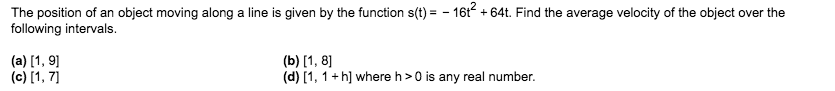# The position of an object moving along a line is given by the function s(t)=-16t64t. Find the average velocity of the object over thefollowing intervals.(a) 1, 9(c) [1, 7(b) 1, 8(d) [1, 1 h] where h>0 is any real number

Question
3 viewshelp_outlineImage TranscriptioncloseThe position of an object moving along a line is given by the function s(t)=-16t64t. Find the average velocity of the object over the following intervals. (a) 1, 9 (c) [1, 7 (b) 1, 8 (d) [1, 1 h] where h>0 is any real number fullscreen
check_circle

Step 1

Since we only answer up to 3 sub-parts, we’ll answer the first 3. Please resubmit the question and specify the other subparts (up to 3) you’d like answered.

We have to use average velocity formula: (s(t2)-s(t1))/(t2-t1)

Step 2

(a) t1=1 and t2=9

Then we plug them in the formula.

Step 3

(b) Here t1=1 and t2=8

Then we plug them in ...

### Want to see the full answer?

See Solution

#### Want to see this answer and more?

Solutions are written by subject experts who are available 24/7. Questions are typically answered within 1 hour.*

See Solution
*Response times may vary by subject and question.
Tagged in

### Other